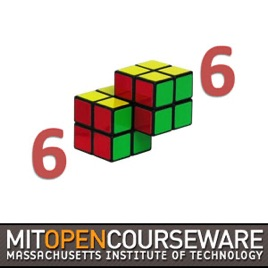47エピソード

Lecture videos from 6.006 Introduction to Algorithms, taught by Erik Demaine and Srini Devadas. The course is divided into eight units: introduction, sorting and trees, hashing, numerics, graphs, shortest paths, dynamic programming, and advanced topics.Introduction to Algorithms (2011) MIT

• テクノロジー

Lecture videos from 6.006 Introduction to Algorithms, taught by Erik Demaine and Srini Devadas. The course is divided into eight units: introduction, sorting and trees, hashing, numerics, graphs, shortest paths, dynamic programming, and advanced topics.

4.0／5
1件の評価

1件の評価